# The Physics of da Vinci and Euler

April 15, 2014 —Comments Off

Today is the birthday of two famous physicists, though that is not how they are commonly remembered.

The first is Leonardo da Vinci, who would turn 562 today. He is best remembered as an artist, but did experiments in physics, architecture, botany, anatomy, and engineering.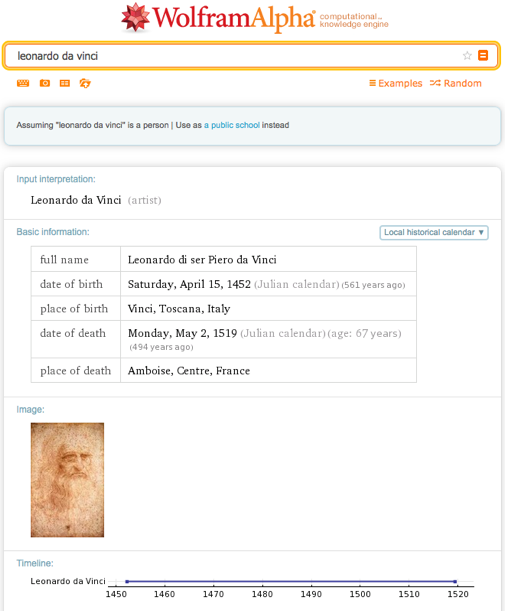The second is Leonhard Euler, who would turn 307 today. He is best remembered as a mathematician, but did experiments in physics, mechanics, astronomy, optics, and music theory. He’s considered one of the greatest mathematicians ever.Due to the huge amount of work they both did, there is some overlap. For example, put a block on an inclined plane, and adjust the angle of the plane until the block starts moving.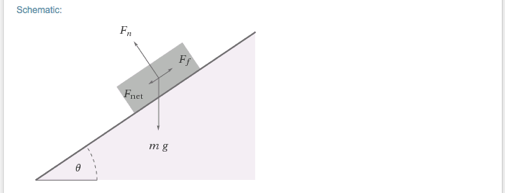Some objects have more friction than others, which will change the angle required. Da Vinci did some of the first known recorded experiments on the topic of sliding friction, and Euler was the first to go into detail on the coefficient of friction.

They both worked on the mechanics of bending a beam. For building objects like Ferris wheels and the Eiffel Tower, knowledge of how beams would behave was vital. Da Vinci did some of the first experiments, and Euler was the first to explain the beam equation in detail.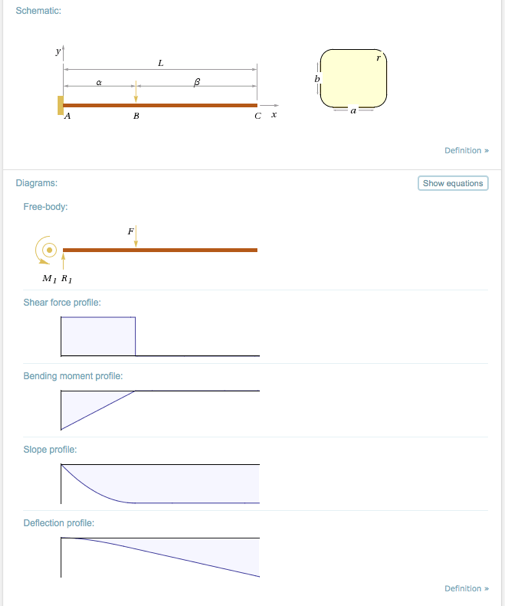Both also worked on polyhedra. Here is something da Vinci called the Duodecedron Abscisus Elevatus Vacuus.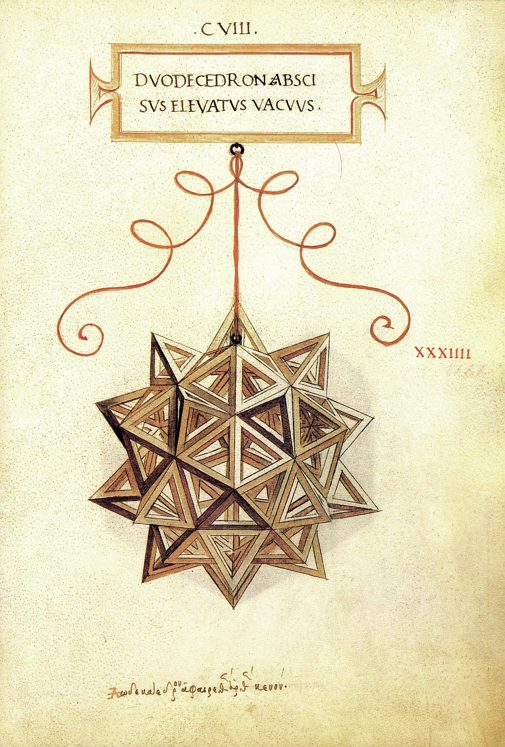We can build up to this with the cumulated icosahedron, or Spikey. With cumulation, each face is replaced with a pyramid of equilateral triangles. Da Vinci’s object could be called a cumulated icosidodecahedron.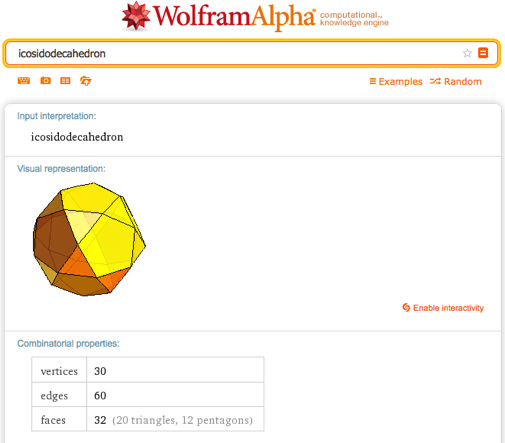With 20 triangles and 12 pentagons, that would need 20*3 + 12*5 = 120 triangles. Each edge touches 2 triangles, so there are 120*3 / 2 = 180 edges. How many vertices are there? You could count, but it’s easier to use Leonhard’s polyhedral formula, which is vertices (V) equals 2 plus edges (E) minus faces (F). V = 2 + EF. Or 62 = 2 + 180 – 120.Happy birthday, da Vinci and Euler.